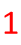# Primes and Composites

There are many ways to categorize numbers. Some people group them in odds and evens or by the number of digits. We can also place numbers in groups by being either prime or composite. First, let's take a look at what these two words mean.
Prime: A number with exactly two factors; one and itself.

What does this mean? This means that for us to call a number prime, only two whole numbers can divide into it without a remainder.

Here are a few examples: 2, 3, 5, 7, 11, 13, 17, 19, 23,...

There are an infinite number of primes. The list could go on and on.

The only whole numbers that divide evenly into 2 are 1 and 2.

The only whole numbers that divide evenly into 3 are 1 and 3.

The only whole numbers that divide evenly into 5 are 1 and 5.

We could keep on going, but we would never be done with the list of primes, so let's move on to Composites.

Composite:A number with more than two factors.

This means that something other than 1 and itself must divide evenly into the composite number.

Here are a few examples: 4, 6, 8, 9, 10, 12, 14, 15, 16, 18, 20, 21, 22, 24, 25, 26, 27, 28,...

There are also an infinite number of composites. This list could also go on and on.

The factors of 4 are 1, 2, 4. Notice that there is something in that list other than 1 or 4!

The factors of 6 are 1, 2, 3, 6. Notice again that there are things other than 1 and 6 in that list.

As soon as you can list a third factor, you have a composite number!

Did you notice that a certain number is missing?

Where is?

1 is not prime or composite. It only has 1 factor -- itself! You could say that 1 is the loneliest number. It does not fit in either category. It does not have exactly two factors to be a prime and it also does not have more than two factors to be with the composites. So if you are even asked if 1 is prime or composite, you should answer, neither!

Common Misconceptions:

All the odds are prime.

This is FALSE! Some odds are prime, like 3, 7, 11...

However, there are primes that have more than 2 factors, like 9. The factors of 9 are 1, 3, and 9. Because there are more than 2 factors, 9 is composite.

All evens are composite.

This is also FALSE. Most evens are composite. However, there is one even that is prime. This would be 2. The only factors of 2 are 1 and 2.

Numbers that end in 1 and 7 are prime.

This is also FALSE. Although there are some numbers that end in 1 or 7 that are prime (like 11, 17, 31, and 37), there are also composite numbers that end in 1 and 7.

These numbers are often called "fake primes" because they look prime, but they aren't. Here are some examples: 51, 57, 81, 87, 91.

Let's take a look at why they are composite.

Factors of 51: 1, 3, 17, 51

Factors of 57: 1, 3, 19, 57

Factors of 81: 1, 3, 9, 9, 27, 81

Factors of 87: 1, 3, 29, 87

Each of these have a factor of 3. So when you are not sure if you have a true prime or a faker, you can see if the number is divisible by 3 to help you figure it out.

Factors of 91: 1, 7, 13, 91

When three doesn't work, you should also try 7 to get rid of more fake primes.

Let's Review:Prime numbers have exactly two factors: 1 and itself. Composites have more than two factors. The number 1 is neither prime nor composite. Finally, if you are trying to figure out if an odd number is a prime, test to see if the number is divisible by primes like 3 and 7 first. This will help you get rid of most of the fake primes.